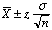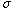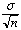Subject: statistics Name: John Barekman Level: secondary I am a student Statitistics: Estimating the population mean when the standard deviation is known: I am not sure which n to use in the formula for the confidence interval equation: x +/- z*(standard deviation/sqrt(n)) If we have data of ten people, and if we have the data of ten sets of ten people each, what is the difference in the n that we use? What is the difference between the standard deviation and the standard error? Are we using the number of sampling means or just the number of samples? Hi John, In the confidence expression that you haven designates the sample size. The value that you use for n is determined by the exact question you are trying to answer. I am assuming that you have ten sets of ten people and you are to construct ten confidence intervals. If this is the case then for each of the confidence intervals the sample size is ten, so n = 10 in each case.    The standard deviation is the quantity usually designatedand the standard error of the maen is. Cheers, Harley

Go to Math Central